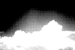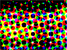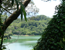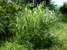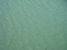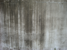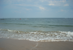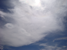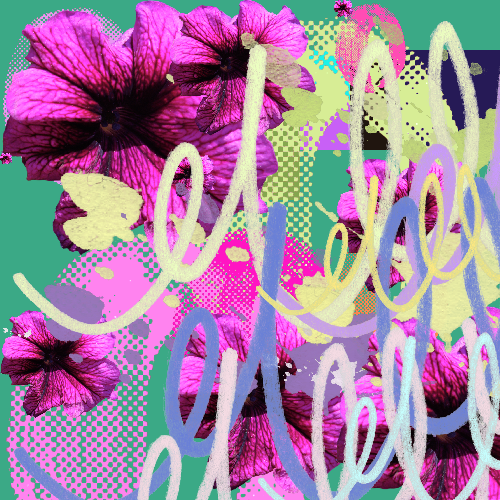###ADD LAYERS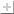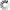•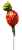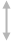per row
Randomize scaling
Base size

Randomize color
Pixelate
Size

•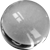per row
Randomize scaling
Base size

Randomize color
Pixelate
Size

•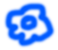per row
Randomize scaling
Base size

Randomize color
Pixelate
Size

•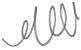per row
Randomize scaling
Base size

Randomize color
Pixelate
Size

•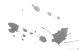per row
Randomize scaling
Base size

Randomize color
Pixelate
Size

•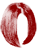per row
Randomize scaling
Base size

Randomize color
Pixelate
Size

•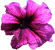per row
Randomize scaling
Base size

Randomize color
Pixelate
Size

•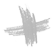per row
Randomize scaling
Base size

Randomize color
Pixelate
Size

•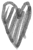per row
Randomize scaling
Base size

Randomize color
Pixelate
Size

•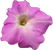per row
Randomize scaling
Base size

Randomize color
Pixelate
Size

•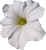per row
Randomize scaling
Base size

Randomize color
Pixelate
Size

•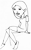per row
Randomize scaling
Base size

Randomize color
Pixelate
Size

•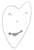per row
Randomize scaling
Base size

Randomize color
Pixelate
Size

•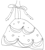per row
Randomize scaling
Base size

Randomize color
Pixelate
Size

•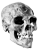per row
Randomize scaling
Base size

Randomize color
Pixelate
Size

•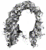per row
Randomize scaling
Base size

Randomize color
Pixelate
Size

•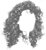per row
Randomize scaling
Base size

Randomize color
Pixelate
Size

•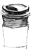per row
Randomize scaling
Base size

Randomize color
Pixelate
Size

•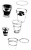per row
Randomize scaling
Base size

Randomize color
Pixelate
Size

•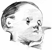per row
Randomize scaling
Base size

Randomize color
Pixelate
Size

•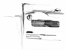per row
Randomize scaling
Base size

Randomize color
Pixelate
Size

Tile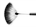per row
Randomize tile size
Amount
Randomize tile position
Amount
Pixelate
Pixel size
Transparency
Overlay filter
Set Color
Monochrome
ImagePixelate
Pixel size
Grayscale